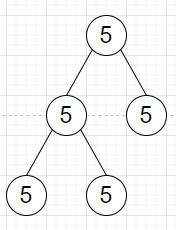# Program to check all values in the tree are same or not in Python

Suppose we have a binary tree, we have to check whether all nodes in the tree have the same values or not.

So, if the input is likethen the output will be True

To solve this, we will follow these steps −

• Define a function solve() . This will take root, and val

• if root is null, then

• return True

• if val is not defined, then

• val := value of root

• return true when value of root is same as val and solve(left of root, val) and solve(right of root, val) are also true

Let us see the following implementation to get better understanding −

## Example

Live Demo

class TreeNode:
def __init__(self, val, left=None, right=None):
self.val = val
self.left = left
self.right = right
class Solution:
def solve(self, root, val=None):
if not root:
return True
if val is None:
val = root.val
return root.val == val and self.solve(root.left, val) and
self.solve(root.right, val)
ob = Solution()
root = TreeNode(5)
root.left = TreeNode(5)
root.right = TreeNode(5)
root.left.left = TreeNode(5)
root.left.right = TreeNode(5)
print(ob.solve(root))

## Input

root = TreeNode(5)
root.left = TreeNode(5)
root.right = TreeNode(5)
root.left.left = TreeNode(5)
root.left.right = TreeNode(5)

## Output

True